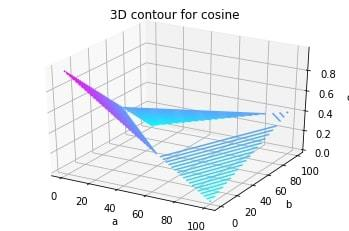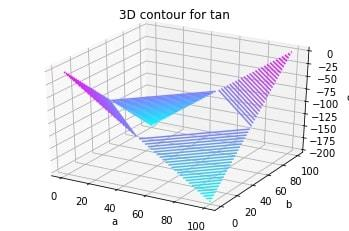Open in App
Not now

# 3D Contour Plotting in Python using Matplotlib

• Last Updated : 21 Apr, 2020

Matplotlib was introduced keeping in mind, only two-dimensional plotting. But at the time when the release of 1.0 occurred, the 3d utilities were developed upon the 2d and thus, we have 3d implementation of data available today! The 3d plots are enabled by importing the mplot3d toolkit. Let’s look at a 3d contour diagram of a 3d cosine function. The code is attached for reference.

Function Arguments Description
meshgrid Function of numpy used to create a rectangular grid out of two given one-dimensional arrays representing the Cartesian indexing or Matrix indexing
plt.axes() To create object of the axis
ax.contour3D To form the contour
ax.set_xlabel To label the X-axis
ax.set_title To give a title to the plot

Example 1:

 `from` `mpl_toolkits ``import` `mplot3d ` `import` `numpy as np ` `import` `matplotlib.pyplot as plt ` `from` `matplotlib ``import` `cm ` `import` `math ` ` `  `x ``=` `[i ``for` `i ``in` `range``(``0``, ``200``, ``100``)] ` `y ``=` `[i ``for` `i ``in` `range``(``0``, ``200``, ``100``)] ` ` `  `X, Y ``=` `np.meshgrid(x, y) ` `Z ``=` `[] ` `for` `i ``in` `x: ` `    ``t ``=` `[] ` `    ``for` `j ``in` `y: ` `        ``t.append(math.cos(math.sqrt(i``*``2``+``j``*``2``))) ` `    ``Z.append(t) ` ` `  `fig ``=` `plt.figure() ` `ax ``=` `plt.axes(projection``=``'3d'``) ` `ax.contour3D(X, Y, Z, ``50``, cmap``=``cm.cool) ` `ax.set_xlabel(``'a'``) ` `ax.set_ylabel(``'b'``) ` `ax.set_zlabel(``'c'``) ` `ax.set_title(``'3D contour for cosine'``) ` `plt.show() `

Output:Example 2: Let’s look at another 3d diagram for better understanding of the concept. This time, of a 3d tan function.

 `from` `mpl_toolkits ``import` `mplot3d ` `import` `numpy as np ` `import` `matplotlib.pyplot as plt ` `from` `matplotlib ``import` `cm ` `import` `math ` ` `  `x ``=` `[i ``for` `i ``in` `range``(``0``, ``200``, ``100``)] ` `y ``=` `[i ``for` `i ``in` `range``(``0``, ``200``, ``100``)] ` ` `  `X, Y ``=` `np.meshgrid(x, y) ` `Z ``=` `[] ` `for` `i ``in` `x: ` `    ``t ``=` `[] ` `    ``for` `j ``in` `y: ` `        ``t.append(math.tan(math.sqrt(i``*``2``+``j``*``2``))) ` `    ``Z.append(t) ` ` `  `fig ``=` `plt.figure() ` `ax ``=` `plt.axes(projection``=``'3d'``) ` `ax.contour3D(X, Y, Z, ``50``, cmap``=``cm.cool) ` `ax.set_xlabel(``'a'``) ` `ax.set_ylabel(``'b'``) ` `ax.set_zlabel(``'c'``) ` `ax.set_title(``'3D contour for tan'``) ` `plt.show() `

Output:My Personal Notes arrow_drop_up
Related Articles